### Home > CALC > Chapter 8 > Lesson 8.3.3 > Problem8-127

8-127.
1. Multiple Choice: If x2 + y2 = 25 then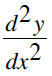= Homework Help ✎

1.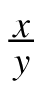2.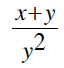3.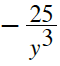4.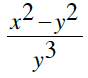5.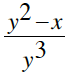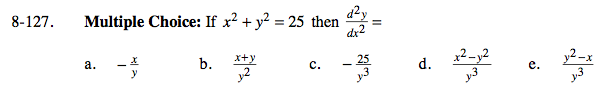$\frac{d}{dx}(x^{2}+y^{2})=\frac{d}{dx}25$

$2x\frac{dx}{dx}+2y\frac{dy}{dx}=0$

$\frac{dy}{dx}=\frac{-x}{y}$

$\frac{d^{2}y}{dx^{2}}=\frac{d}{dx}\left (\frac{-x}{y} \right )$

$\frac{d^2y}{dx^2}=\frac{-1}{y}\frac{dx}{dx}+\frac{xy}{y^2}\frac{dy}{dx}$

$\frac{d^2y}{dx^2}=\frac{-1}{y}+\frac{xy}{y^2}\cdot\frac{-x}{y}$

$\frac{d^{2}y}{dx^{2}}=\frac{-(y^{2}+x^{2})}{y^{3}}$

Uh-oh! None of the multiple choice options match! That does not mean you are wrong. Look at the original equation of the circle. What does x² + y² equal? Substitute that value and find your solution.